\clearpage

This vignette describes how the R package rehh can be applied to perform whole genome scans for footprints of selection using statistics related to Extended Haplotype Homozygosity (EHH) [@Sabeti2002].

The package accepts multi-allelic genetic markers as input. Typically, albeit not necessarily, these will be bi-allelic SNPs.

The package is available for Linux, Windows and MacOS X from the CRAN repository https://cran.r-project.org/package=rehh and may be installed using a standard procedure. Once the package has been successfully installed, it can be loaded by:

library(rehh)


## Background

The analysis of molecular population genetic data often comprises the search for genomic regions that might have experienced recent selection. Diverse approaches have been developed, reviewed e.g. in [@Oleksyk2010] and [@Vitti2013], however only a few have found wide-spread application [@Cadzow2014], [@Haasl2016]. To the latter belong iHS [Voight2006], Rsb [@Tang2007] and XP-EHH [@Sabeti2007], all of which are summary statistics aimed to distill a certain aspect of the genetic data into a single score and constructed in a way that extreme values are indicative of positive or “Darwinian” selection. iHS is intended for application on a single (presumably homogeneous) population, while XP-EHH and Rsb are targeted to differential selection between two populations. All three statistics are based on the concept of Extended Haplotype Homozygosity (EHH) as formulated by [@Sabeti2002].

iHS and XP-EHH can be calculated by the independent C++ command line tool Hapbin [@Maclean2015], which has been optimized for speed by exploiting bit-wise machine-level operations. The package rehh cannot compete on performance, but has the advantage of being able to work with multi-allelic markers and missing values. Moreover, it possesses a broader range of input and output options, including several graphical representations.

## Changes between versions 2.X and 3.X

The C routines responsible for the bulk of calculations have been rewritten and all of the R code has been largely revised and streamlined yielding the following new features:

• the package accepts multi-allelic markers.
• support for input files in variant call format as well as the output format of the simulation program ms [@Hudson2002].
• computation of statistics and their visualization has become separated into different functions.
• graphical plots are more customizable.
• furcation diagrams can be labeled and a related visualization of “haplotype length” was added.
• a function to define candidate regions of selection.
• adaptations for unphased haplotypes or unpolarized alleles.
• output in form of matrices has been replaced by data frames; some columns have become optional.
• all names of data frames are now in lower case letters while all column names contain exclusively capital letters and underscores.
• a new internal representation of alleles: previously, an ancestral allele was coded by 1, a derived allele by 2 and a missing value by 0. These codings have been replaced by 0, 1 and NA, respectively. Furthermore, the numbers are now explicitly of type “integer” instead of “numeric”.
• a second vignette to explain the statistics involved and the functionality of the package using an invented tiny data set.
• additional options to yield virtually identical results with the program hapbin (see section \@ref(hapbin)).
• an inconsistency between implementation and documentation concerning the calculation of one-sided p-values has been cleared.

Due to changes in the API, although mostly small, the versions 3.X are not compatible with versions 2.X!

Data objects of class haplohh (see below) created by versions up to 2.0.4 must be updated via the command update_haplohh() (see its documentation by typing ?update_haplohh) in order to be accepted by the functions of the current version.

## Example files

For illustration purposes, several example input files as well as R objects are provided.

### Input files

• Two tiny invented examples, each in our “standard” haplotype format (see section \@ref(input)) and in variant call format (vcf). The first example was used in [@Gautier2017] for the explanation of the various statistics calculated by this package. The second example is an extension of the former including multi-allelic markers and missing values. Both sets are discussed in depth in a second vignette.

• An output file of the program ms containing two small simulated haplotype samples.

• Input files in different formats that originate from a study on the “Creole cattle breed from Guadeloupe” (CGU) [@Gautier2011]. All files contain the same set of phased SNPs of Bos taurus chromosome 12 from 140 individuals.

All of the above files are copied into the current working directory via the command

make.example.files()


Throughout the vignette, this command is assumed to have been run!

### R objects

• The data for chromosome 12 of the cattle study mentioned above as object of the rehh data class haplohh. This object becomes available by the command data(haplohh_cgu_bta12).

• The scores iHH and iES as obtained by the function scan_hh() applied on SNPs of the whole genome for the population CGU (defined above) and another population EUT (“European taurine”). They reside in the accompanying package rehh.data and are obtained by library(rehh.data) followed by data(wgscan.cgu) resp. data(wgscan.eut).

## Terminology

Depending on the context, we refer as chromosomes either to the different chromosomes of a species' reference sequence (e.g. “chr1”, “chr2”, “chrX”, etc.) or to the homologous chromosomes from different individuals (e.g. 280 times “chr12” from 140 diploid individuals). The sequence of alleles on a chromosome is referred to as its haplotype and sometimes we use it interchangeably with chromosome. However, if we speak of a shared haplotype, the term is meant more abstractly for the unique sequence of alleles that a group of chromosomes share at each position within a certain region.

## Overview

The package calculates three statistics by the following steps:

• each marker is taken in turn as a “focal marker” around which the extended haplotype homozygosity (EHH) is measured
• EHH is summerized into a number by integration
• two integrals are compared by taking their log ratio (two alleles for within-population or two populations for cross-population statistics)
• the genome-wide distribution of these log-ratios is normalized

Here is a minimal code example for a single population and a single chromossome:

hh <-                  # data input
data2haplohh(
hap_file = "bta12_cgu.hap",
map_file = "map.inp",
chr.name = "12",
allele_coding = "map"
)
scan <- scan_hh(hh)    # calculation of EHH and integration
ihs <- ihh2ihs(scan)   # log ratio for alleles and normalization
manhattanplot(ihs)     # plot of the statistics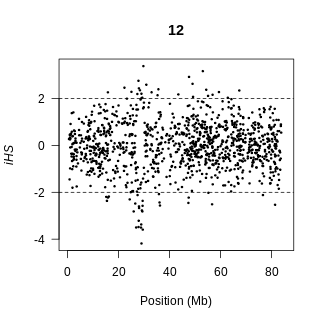# Data input {#input}

The package rehh requires as input:

• a haplotype data file for each population of interest (see section \@ref(hapfile)).

and, if the haplotype data file is neither in variant call format nor in the format of ms output,

• a marker information file (see section \@ref(mapfile)).

Since R can automatically recognize and read compressed files with standard name extensions like .gz or .bzip2, this holds as well for the input files that can be used with this package.

## Haplotype data file {#hapfile}

Five haplotype input file formats are supported:

• a standard haplotype format. Each row represents a haplotype with marker genotype in columns as in the example file bta12_cgu.hap containing 280 haplotypes with 1424 SNPs each (see section \@ref(LoadDataEx1)). The first element of each row is taken as a haplotype identifier.
• a transposed format with haplotypes in columns and markers in rows as in the example file bta12_cgu.thap. This format is similar to the one produced by the phasing program SHAPEIT2 [@OConnell2014]. This format assumes neither row nor column names and hence no haplotype identifiers can be specified (see section \@ref(LoadDataEx2)).
• the output file format from the phasing program fastPHASE [@Scheet2006] as in the bta12_hapguess_switch.out example file. Note that this file format allows to include haplotypes from several populations (if the -u fastPHASE option was used) (see section \@ref(LoadDataEx3)).
• variant call format (vcf), comprising both haplotype and marker information. In order to read files in this format, the package vcfR has to be installed (see section \@ref(LoadDataEx4)).
• The output of the simulation program ms [@Hudson2002] and its derivatives msHOT [@Hellenthal2007], ms2^ [@Ewing2010] and ms' [@Kelleher2016]. In order to read these files, the package gap has to be installed (see section \@ref(LoadDataEx5)).

Alleles in standard or transposed haplotype format can be provided either coded (by integer numbers) or without coding (e.g. as nucleotides) (see section \@ref(LoadData)).

If alleles are not polarized, i.e. their ancestral/derived status is not known, some arbitrary assignation can be done either beforehand by the user or during input by the package; appropriate parameters must be set in subsequent functions (see section \@ref(polarizing)).

## Marker information file {#mapfile}

For haplotype data files in standard, transposed or fastphase format a marker information file has to be provided. The file should contain columns without header corresponding to:

1. marker name/identifier
2. name of the chromosome (or scaffold)
3. position on the chromosome (or scaffold), either physical (in bases) or genetic (e.g. in centiMorgans).

For a given chromosome, the markers must be ordered in the same way as in the haplotype data file.

If alleles in the haplotype file are not already provided in coded form (i.e. as positive integers), the package can (re)code them using two additional columns in the marker file:

1. ancestral allele
2. derived allele(s).

For instance, the provided file map.inp has five columns:

# show first 6 lines of file
cat(readLines("map.inp", n = 6), sep = "\n")

> F0100190 1 113642 T A
> F0100220 1 244699 C G
> F0100250 1 369419 G C
> F0100270 1 447278 A T
> F0100280 1 487654 T A
> F0100290 1 524507 C G


## Loading data files: the function data2haplohh {#LoadData}

The data2haplohh() function converts data files into an R object of class haplohh, forming the basis for subsequent analysis. The following options are available to recode alleles and/or to filter markers and haplotypes:

1. Allele coding options:

The parameter allele_coding has four options: three of them expect the user to provide a coding, while the fourth option overwrites any user defined coding.

The package accepts two different integer codings within the haplotype file which can be specified as "12" or "01", respectively:

“12” “01”
Missing value 0 NA or .
Ancestral allele 1 0
Derived allele 1 2 1
Derived allele 2 3 2

The latter one is also the coding used internally by our package.

If the alleles are given in an un-coded form (e.g. as nucleotides), the alleles can be coded for each marker separately with help of the marker information file. This option is activated by allele_coding = "map". If an allele of a marker is found in the fourth column of the marker information file, it is re-coded as 0 (ancestral) and if it is found in the fifth column it is re-coded as 1 (derived). The fifth column may contain several alleles, separated by commas, like in the variant call format. In this case, the internal coding extends to higher integers. If an allele present in the haplotype file is not found neither in the fourth nor the fifth column of the marker information file, it is counted as NA (missing value).

If the alleles are not polarized, i.e. it is unknown which allele is ancestral and which derived, the option "none" should be selected. The alleles will then be coded in alpha-numeric order for each marker; the first becomes 0, the second 1, etc. Missing values must be given as NA or '.' while any other number, character or string will be counted as an allele. Options of subsequent analysis functions have to be set accordingly (see section \@ref(polarizing)).

2. Filtering options

2.1 Discard haplotypes with a high amount of missing data. If haplotypes contain missing data (presumably a rare case since most phasing programs allow imputing missing genotypes), it is possible to discard those with a fraction of less than min_perc_geno.hap of markers genotyped. Its default is NA, hence no restriction.

2.2. Discard markers with a high amount of missing data. It is possible to discard individual markers genotyped on a fraction of less than min_perc_geno.mrk of haplotypes. By default min_perc_geno.mrk = 100 meaning that only fully genotyped markers are retained. A value of NA or zero is not allowed since rehh cannot handle markers with no data.

2.3 Discard markers with a low Minor Allele Frequency. It is possible to discard markers with a Minor Allele Frequency (MAF) equal to or below min_maf. Its default is NA meaning no constraint. Setting this value to zero eliminates monomorphic markers. Note that since low-frequency alleles usually abound, any positive value may exclude a substantial fraction of the data.

The arguments min_perc_geno.hap, min_perc_geno.mrk and min_maf are evaluated in this order.

More details about input options can be found in the documentation using the command:

?data2haplohh


The following command converts the haplotype input file bta12_cgu.hap (in “standard” haplotype format) and marker information input file map.inp to an haplohh object named hh. Because the map file contains information for markers mapping to multiple chromosomes we have to specify by chr.name = 12 that the haplotype input file is about chromosome 12. Alleles are given as nucleotides in the haplotype file and coded with help of the map file by setting allele_coding = "map".

hh <- data2haplohh(hap_file = "bta12_cgu.hap",
map_file = "map.inp",
chr.name = 12,
allele_coding = "map")

> * Reading input file(s) *
> Map info: 1424 markers declared for chromosome 12 .
> Haplotype input file in standard format assumed.
> Alleles are being recoded according to fourth and fifth column of map file.
> * Filtering data *
> Discard markers genotyped on less than 100 % of haplotypes.
> Data consists of 280 haplotypes and 1424 markers.
> Number of mono-, bi-, multi-allelic markers:
> 1 2
> 27 1397


If no value is specified for chr.name and more than one chromosome is detected in the map file, an error is produced:

hh <- data2haplohh(hap_file = "bta12_cgu.hap",
map_file = "map.inp",
allele_coding = "map")

> * Reading input file(s) *
> More than one chromosome name in file: map.inp .
> Here is a list of chromosome names found:
>  1 2 3 4 5 6 7 8 9 10 11 12 13 14 15 16 17 18 19 20 21 22 23 24 25 26 27 28 29

> Error: Please specify a chromosome name. Conversion stopped.


Furthermore, the following message is prompted if the number of markers for the chromosome in the info file does not correspond to the one in the haplotype file (for instance when a wrong chromosome is specified):

hh <- data2haplohh(hap_file = "bta12_cgu.hap",
map_file = "map.inp",
chr.name = 18,
allele_coding = "map")

> * Reading input file(s) *
> Map info: 1123 markers declared for chromosome 18 .
> Haplotype input file in standard format assumed.

> Error: The number of markers in the haplotype file (1424) is not equal
> to the number of markers in the map file (1123).
> Conversion stopped.


If the haplotype input file bta12_cgu.thap is in “transposed” format, the option haplotype.in.columns has to be set to TRUE while all other parameters remain unaffected with respect to example 1. Note that this is the only format that has to be explicitly declared by the user.

hh <- data2haplohh(hap_file = "bta12_cgu.thap",
map_file = "map.inp",
chr.name = 12,
allele_coding = "map",
haplotype.in.columns = TRUE)

> * Reading input file(s) *
> Map info: 1424 markers declared for chromosome 12 .
> Haplotype input file in transposed format assumed.
> Alleles are being recoded according to fourth and fifth column of map file.
> * Filtering data *
> Discard markers genotyped on less than 100 % of haplotypes.
> Data consists of 280 haplotypes and 1424 markers.
> Number of mono-, bi-, multi-allelic markers:
> 1 2
> 27 1397


In this example, we use the fastPHASE output bta12_hapguess_switch.out as haplotype file. This format is recognized automatically. As before, we need to set chr.name = 12. Because haplotypes originated here from several populations (the fastPHASE option -u was used), it is necessary to select the population of interest (in our case “CGU”) by its corresponding number in the file, hence popsel = 7.

hh <- data2haplohh(hap_file = "bta12_hapguess_switch.out",
map_file = "map.inp",
chr.name = 12,
popsel = 7,
allele_coding = "map")

> * Reading input file(s) *
> Map info: 1424 markers declared for chromosome 12 .
> Haplotype input file in fastPHASE format assumed.
> Haplotypes in the fastPHASE output file originate from 8 populations.
> Alleles are being recoded according to fourth and fifth column of map file.
> * Filtering data *
> Discard markers genotyped on less than 100 % of haplotypes.
> Data consists of 280 haplotypes and 1424 markers.
> Number of mono-, bi-, multi-allelic markers:
> 1 2
> 27 1397


If no value is specified for the popsel argument and more than one population is detected in the fastPHASE output file, an error in produced and the available population numbers printed:

hh <- data2haplohh(hap_file = "bta12_hapguess_switch.out",
map_file = "map.inp",
chr.name = 12,
allele_coding = "map")

> * Reading input file(s) *
> Map info: 1424 markers declared for chromosome 12 .
> Haplotype input file in fastPHASE format assumed.
> Haplotypes in the fastPHASE output file originate from 8 populations.

> Error: Please specify by 'popsel' one of the following population numbers:
> 1 2 3 4 5 6 7 8
> Conversion stopped.


The function data2haplohh() checks automatically whether the specified haplotype input file is in variant call format (vcf). If this is the case, the parameters map_file and allele_coding are ignored. By default, the function tries to polarize (see section \@ref(polarizing)) the alleles of each marker using the ancestral allele, expected to be given by key AA of the INFO field.

Ancestral alleles are sometimes marked by upper case as “high confident” and by lower case as “low confident”. The default setting capitalize_AA = TRUE lifts this distinction before polarization.

If the AA key is absent, the option polarize_vcf should be set to FALSE and the allele coding of the vcf file is directly used as internal coding.

If there is data for more than one chromosome in the file, the chromosome of interest has to be specified by chr.name. Since always the whole file is read in, it may be advisable for large data sets to create chromosome-specific files.

In order to process vcf files, the package vcfR has to be installed.

In the file bta12_cgu.vcf.gz the ancestral allele was set as reference and hence no further polarizing is necessary.

hh <- data2haplohh(hap_file = "bta12_cgu.vcf.gz",
polarize_vcf = FALSE)

> * Reading input file(s) *
> Scanning file to determine attributes.
> File attributes:
>   meta lines: 4
>   variant count: 1424
>   column count: 149
>
> All meta lines processed.
> gt matrix initialized.
> Character matrix gt created.
>   Character matrix gt rows: 1424
>   Character matrix gt cols: 149
>   skip: 0
>   nrows: 1424
>   row_num: 0
>
Processed variant 1000
Processed variant: 1424
> All variants processed
> Extracting map information.
> Extracting haplotypes.
> Number of individuals which are
> Haploid Diploid Triploid, ... :
> 1 2
> 0 140
> * Filtering data *
> Discard markers genotyped on less than 100 % of haplotypes.
> Data consists of 280 haplotypes and 1424 markers.
> Number of mono-, bi-, multi-allelic markers:
> 1 2
> 27 1397


The function data2haplohh() automatically checks whether the haplotype file is in the output format of the simulation program ms [@Hudson2002]. If this is the case, the parameters map_file and allele_coding are ignored. If the file contains several 'runs' (as referred to by the parameter nrep of ms), it is necessary to specify the number of the run in option chr.name. Note that always the whole file is read, so that it might be advisable to spread large simulations over separate files.

One argument of the data2haplohh function is specifically dedicated to ms output, although it works with other formats as well: ms gives chromosomal positions as fractions of the interval [0,1] and in order to obtain more realistic values, these positions can be multiplied by a factor, set by position_scaling_factor.

Note that rehh does not accept multiple markers with the same position and hence it is highly recommended to increase the numerical precision for chromosomal positions in the ms output.

In order to read this format, the package gap has to be installed.

hh <- data2haplohh(hap_file = "ms.out",
chr.name = 2,
position_scaling_factor = 1000)

> * Reading input file(s) *
> Input file in 'ms' output format assumed.
>
> Extracting positions.
> Extracting haplotypes.
> * Filtering data *
> Discard markers genotyped on less than 100 % of haplotypes.
> Data consists of 10 haplotypes and 39 markers.
> Number of mono-, bi-, multi-allelic markers:
> 1 2
> 0 39


## Subset data

Sometimes it might be of interest to restrict the computation of statistics to subsets of haplotypes or markers. This can be done with help of the function subset() and arguments select.hap and select.mrk. After subsetting, the same filters are applied as during data input from files in order to ensure that no marker has exclusively missing values and, optionally, to exclude markers which are monomorphic within the subset by setting min_maf to zero.

For example, to restrict the output of ms (see section \@ref(LoadDataEx5)) to the first five haplotypes and filter out monomorphic sites, we apply

hh_subset = subset(hh, select.hap = 1:5, min_maf = 0)

> * Subset haplotypes *
> 5 haplotypes remaining.
> * Filtering data *
> Discard markers genotyped on less than 100 % of haplotypes.
> Discard markers with Minor Allele Frequency equal to or below 0 .
> 9 markers remaining.
> Data consists of 5 haplotypes and 9 markers.
> Number of mono-, bi-, multi-allelic markers:
> 1 2
> 0 9


while the following command omits the first marker:

hh_subset = subset(hh, select.mrk = -1)

> * Subset markers *
> 38 markers remaining.
> * Filtering data *
> Discard markers genotyped on less than 100 % of haplotypes.
> Data consists of 10 haplotypes and 38 markers.
> Number of mono-, bi-, multi-allelic markers:
> 1 2
> 0 38


# Computing EHH, EHHS and their “integrals” iHH and iES

## Definition and computation

### The (allele-specific) Extended Haplotype Homozygosity (EHH) {#EHH}

For any given allele $$a$$ of a focal marker $$s$$, sometimes referred to as a core allele, the Extended Haplotype Homozygosity (EHH) is defined as the probability that two randomly chosen chromosomes, carrying the core allele, are homozygous over a given surrounding chromosomal region [@Sabeti2002]. It is estimated from a sample by calculating the homozygosity of the chromosomal chunk between the focal marker and another marker $$t$$ by the formula \begin{equation} \mathrm{EHH}{s,t}a=\frac{1}{n{a}(na-1)}\sum\limits{k=1}{Ka_{s,t}}n_k(n_k-1) (#eq:ehh) \end{equation} where $$n_a$$ represents the number of chromosomes carrying the core allele $$a$$, $$K^a_{s,t}$$ represents the number of different shared haplotypes and $$n_k$$ refers to the number of chromosomes pertaining to the $$k$$-th such shared haplotype. If there is no missing data, it holds that $$n_a=\sum\limits_{k=1}^{K^a_{s,t}}n_k$$.

In the case of unphased chromosomes from diploid individuals (see section \@ref(phasing)) the extended haplotype homozygosity can be calculated as follows [@Tang2007]: we consider only chromosomes from individuals that are homozygous for the allele $$a$$ at the focal marker $$s$$ and estimate EHH at some marker $$t$$ by the fraction of individuals that are (still) homozygous over the entire chromosomal stretch between $$s$$ and $$t$$. Let $$I^a_{s,t}$$ denote the number of individuals that are homozygous from marker $$s$$ til marker $$t$$. We can reformulate the fraction of individuals in terms of the fraction of shared haplotypes: since haplotypes of different individuals are not compared they can be regarded as distinct by definition, hence $$K_{s,s}^a=I_{s,s}^a=\frac{1}{2}n_a$$. With increasing distance of $$t$$ from $$s$$, any increase in $$K_{s,t}^a$$ is tantamount to a decrease of the number of homozygous individuals, yielding \begin{equation} \mathrm{EHH}{s,t}a=\frac{I{s,t}a}{I{s,s}a}=\frac{n_a-K{s,t}a}{\frac{1}{2}n_a}\;. (#eq:ehh2) \end{equation}

No matter which of the two definitions is used, it is common practice to stop computation when EHH reaches a certain lower threshold, e.g. 0.05.

### The integrated EHH (iHH) {#iHH}

By definition, irrespective of the allele considered, EHH starts at 1 and decays to 0 with increasing distance of t from the focal marker s. For a given core allele, the integrated EHH (iHH) is defined as the area under the EHH curve which, in turn, is defined by the EHH values and associated chromosomal positions [@Voight2006]. The integral is computed with a simple standard method, called the trapezoidal rule.

### The (site-specific) Extended Haplotype Homozygosity (EHHS) {#EHHS}

An extended haplotype homozygosity can be defined as well without reference to core alleles. In this case, the quantity is aimed to reflect the probability that any two randomly chosen chromosomes from a population are homozygous over a given surrounding chromosomal region of a focal marker. In contrast to the allele-specific EHH defined in the previous section, the chromosomes are not required to carry a specific allele at the focal marker. We adopt the naming by [@Tang2007] as site–specific EHH, abbreviated by EHHS. Note, however that this quantity is sometimes referred to as EHH, too, and there is no agreed notation in the literature.

EHHS was used in genome scans in two versions: un-normalized by [@Sabeti2007] and normalized by [@Tang2007].

In line with [@Sabeti2007] we define \begin{equation} (#eq:ehhssab) \mathrm{EHHS}{s,t}=\frac{1}{n_s(n_s-1)}\sum\limits{k=1}{K_{s,t}}n_k(n_k-1) \end{equation} where we re-use notation from above and let $$n_s$$ refer to the number of chromosomes at marker $$s$$. If there are no missing values at that marker, this is simply the number of chromosomes in the sample.

[@Tang2007] proposed an apparently different estimator for the normalized EHHS, which we abbreviate to nEHHS, namely \begin{equation} (#eq:ehhstang) \mathrm{nEHHS}{s,t}=\frac{1-h{s,t}}{1-h_s} \end{equation} where:

1. $$h_s=\frac{n_s}{n_s-1}\left(1-\frac{1}{n_s^2}\left(\sum\limits_{k=1}^{K_{s,s}}n_k^2 \right )\right)$$ is an estimator of the focal marker heterozygosity. In the bi-allelic case we can write this as $$h_s=\frac{n_s}{n_s-1}\left(1-\frac{1}{n_s^2}\left(n_{a1}^2+n_{a2}^2\right) \right)$$ with $$a1$$ and $$a2$$ referring to the numbers of the two alleles at marker $$s$$ ($$n_{a1}+n_{a2}=n_s$$).

2. $$h_{s,t}=\frac{n_s}{n_s-1}\left(1-\frac{1}{n_s^2}\left(\sum\limits_{k=1}^{K_{s,t}}n_k^2 \right )\right)$$ is an estimator of haplotype heterozygosity in the chromosomal region extending from marker $$s$$ to marker $$t$$.

However both definitions are in fact equivalent, because it holds $$\mathrm{EHHS}_{s,t}=1-h_{s,t}$$ and hence \begin{equation} \mathrm{nEHHS}{s,t}=\frac{\mathrm{EHHS}{s,t}}{\mathrm{EHHS}_{s,s}}\;. \end{equation} Thus $$\mathrm{nEHHS}_{s,t}$$ is just normalized in order to yield 1 at the focal marker $$s$$. Note that the normalization factor depends on the frequency of the alleles at the focal marker and consequently is in general not the same for different focal markers.

Furthermore, we note that EHHS and EHH are related by \begin{equation} \mathrm{EHHS}{s,t}=\frac{n{a1}(n{a1}-1)}{n_s(n_s-1)}\mathrm{EHH}{s,t}{a1}+\frac{n{a2}(n{a2}-1)}{ns(n_s-1)}\mathrm{EHH}{a2}{s,t}\;, \end{equation} where for the sake of simplicity we assume that the focal marker has only two alleles $$a1$$ and $$a2$$. EHHS might hence be viewed as a linear combination of the EHH's of the focal alleles, weighted by roughly the square of the focal allele frequencies.

In the case of unphased chromosomes from diploid individuals (see section \@ref(phasing)) EHHS can be calculated like EHH using equation \@ref(eq:ehh2) without the restriction to core alleles: \begin{equation} \mathrm{EHHS}{s,t}=\frac{I{s,t}}{I{s,s}}=\frac{n-K{s,t}}{\frac{1}{2}n}\;. (#eq:ehhs2) \end{equation} Note that defined this way, EHHS is always 1 at the focal marker. Hence there is no distinction between $$\mathrm{EHHS}$$ and $$\mathrm{nEHHS}$$.

Again, unphased EHHS can be related to unphased EHH by \begin{equation} \mathrm{EHHS}{s,t}=\frac{I{s,t}}{I{s,s}}=\frac{I{s,t}{a1}+I{s,t}{a2}}{I{s,s}{a1}+I{s,s}{a2}}=\frac{I{s,s}{a1}}{I{s,s}{a1}+I{s,s}{a2}}\mathrm{EHH}{s,t}{a1}+\frac{I{s,s}{a2}}{I{s,s}{a1}+I{s,s}{a2}}\mathrm{EHH}_{s,t}{a2}\; \end{equation} where for the sake of simplicity we assumed a bi-allelic focal marker with alleles $$a1$$ and $$a2$$.

As with EHH, the EHHS is usually computed only for the region where its value surpasses a given threshold (e.g., EHHS>0.05).

### The integrated EHHS (iES) {#iES}

Like EHH, EHHS has its maximum at the focal marker and decays to 0 with increasing distance from the focal marker. For a given focal marker, analogously to iHH, iES is defined as the integrated EHHS [@Tang2007]. Depending on whether EHHS or nEHHS is integrated, we yield iES and inES respectively. As with iHH, the numerical integration uses the trapezoidal rule.

## The function calc_ehh() {#calcehh}

The function calc_ehh() computes EHH for all alleles of a focal marker $$s$$ relative to markers $$t$$ upstream and downstream. For each allele the corresponding integral iHH of the EHH curve is calculated as well.

Two options can be specified to constrain the computation of EHH. The first, limehh sets a threshold below which further calculation of EHH is stopped. The second, limhaplo defines the smallest acceptable number of evaluated chromosomes. This number decreases at subsequent markers with missing values. The default values of these two conditions are 0.05 and 2, respectively.

By default, the option include_zero_values is FALSE and the output contains only EHH values for markers up to the two thresholds. If the parameter is toggled to TRUE, then values for all markers are returned, the remaining ones being zero.

If calculation reaches the border of the chromosome, i.e. the first or last marker, or a large gap (see below), the integral iHH is discarded in order to avoid boundary effects. This behavior can be turned off by setting discard_integration_at_border to FALSE.

To account for gaps between consecutive markers, two parameters can be set (see also section \@ref(gaps)): scalegap specifies a distance such that any greater gap is re-scaled (or capped) to that size. The option maxgap can be used to stop integration at gaps that are greater than the specified value.

Furthermore, integration is performed by default over the area between the graph defined by the EHH values and the line y = limehh. If numerical agreement with the program Hapbin is wanted, this area should be extended to the x-axis by setting lower_y_bound to zero.

The option polarized, TRUE by default, merely affects the order and labeling of alleles.

Finally, the parameter phased can be toggled to FALSE in order to calculate EHH by the formula for unphased data (see section \@ref(EHH) and \@ref(phasing)).

More details are available in the R documentation by using the command:

?calc_ehh


In the following example, EHH is computed around the SNP F1205400. This SNP is associated with a strong signal of selection (using both iHS and Rsb statistics) and is located closely (<5kb) to a strong candidate gene involved in horn development [@Gautier2011].

Note that the R object haplohh_cgu_bta12 was generated using the data2haplohh() function with the example input files (see section \@ref(LoadDataEx1)).

#example haplohh object (280 haplotypes, 1424 SNPs) see ?haplohh_cgu_bta12 for details
data(haplohh_cgu_bta12)
#computing EHH statistics for the focal SNP with name "F1205400"
#which displays a strong signal of selection
res <- calc_ehh(haplohh_cgu_bta12,
mrk = "F1205400",
include_nhaplo = TRUE)


The output contained in res is a list with four elements:

1. mrk.name: the name/identifier of the focal marker.
2. freq: a vector containing the frequencies of all alleles of the focal marker.
3. ehh: a data frame containing the EHH values for each allele of the focal marker. Setting the parameter include_nhap to TRUE adds columns which show how many chromosomes contributed at each position to the calculation of EHH.
4. ihh: a vector with iHH for each allele, i.e. the integrals over the EHH curves defined by the EHH values.
res$mrk.name  >  "F1205400"  res$freq

>    FREQ_A    FREQ_D
> 0.3035714 0.6964286

res$ehh  > POSITION EHH_A EHH_D NHAPLO_A NHAPLO_D > F1204760 25629699 0.00000000 0.05175786 0 195 > F1204770 25680969 0.00000000 0.05175786 0 195 > F1204780 25727883 0.00000000 0.05207507 0 195 > F1204790 25769786 0.00000000 0.05239228 0 195 > F1204800 25884193 0.00000000 0.05239228 0 195 > F1204810 25932431 0.00000000 0.05249802 0 195 > F1204840 26132031 0.00000000 0.05884219 0 195 > F1204850 26189286 0.00000000 0.05884219 0 195 > F1204870 26255986 0.00000000 0.05884219 0 195 > F1204880 26313792 0.00000000 0.05889506 0 195 > F1204900 26479781 0.00000000 0.05926513 0 195 > F1204910 26507987 0.00000000 0.05926513 0 195 > F1204920 26526646 0.00000000 0.05926513 0 195 > F1204930 26558947 0.00000000 0.06650806 0 195 > F1204940 26599290 0.00000000 0.08675654 0 195 > F1204950 26683271 0.00000000 0.08675654 0 195 > F1204960 26727974 0.00000000 0.11134021 0 195 > F1204970 26779948 0.00000000 0.12513878 0 195 > F1204980 26800201 0.00000000 0.12778218 0 195 > F1204990 26854850 0.00000000 0.13068993 0 195 > F1205000 26902773 0.00000000 0.13967750 0 195 > F1205010 26952729 0.00000000 0.25424266 0 195 > F1205020 26997449 0.00000000 0.37520486 0 195 > F1205030 27031612 0.00000000 0.42008988 0 195 > F1205040 27058533 0.00000000 0.42024848 0 195 > F1205050 27097980 0.00000000 0.47269363 0 195 > F1205070 27200291 0.00000000 0.47269363 0 195 > F1205080 27356111 0.00000000 0.51578113 0 195 > F1205090 27378189 0.00000000 0.52307692 0 195 > F1205100 27465862 0.00000000 0.52307692 0 195 > F1205110 27501592 0.00000000 0.52312979 0 195 > F1205120 27525360 0.00000000 0.53782712 0 195 > F1205130 27588853 0.00000000 0.53782712 0 195 > F1205150 27735666 0.00000000 0.53782712 0 195 > F1205160 27804138 0.00000000 0.54290246 0 195 > F1205170 27833642 0.00000000 0.56674597 0 195 > F1205180 27907564 0.00000000 0.78752313 0 195 > F1205190 27985596 0.00000000 0.80586836 0 195 > F1205200 28014498 0.00000000 0.80602696 0 195 > F1205210 28054852 0.00000000 0.81527888 0 195 > F1205230 28266218 0.00000000 0.85249802 0 195 > F1205240 28296968 0.00000000 0.85249802 0 195 > F1205250 28386447 0.00000000 0.85249802 85 195 > F1205260 28426219 0.05854342 0.90023791 85 195 > F1205270 28562210 0.06694678 0.90023791 85 195 > F1205280 28606777 0.06750700 0.90023791 85 195 > F1205290 28633388 0.08767507 0.91979910 85 195 > F1205300 28689805 0.13137255 0.95945017 85 195 > F1205310 28721292 0.14649860 0.95945017 85 195 > F1205320 28753422 0.16246499 0.95945017 85 195 > F1205330 28786001 0.16246499 0.95945017 85 195 > F1205340 28810078 0.16246499 0.95945017 85 195 > F1205360 28896769 0.16610644 0.98974359 85 195 > F1205370 28925117 0.19103641 1.00000000 85 195 > F1205380 28947722 0.27647059 1.00000000 85 195 > F1205390 28967990 0.55294118 1.00000000 85 195 > F1205400 28993983 1.00000000 1.00000000 85 195 > F1205420 29101326 0.88795518 1.00000000 85 195 > F1205440 29147373 0.64229692 1.00000000 85 195 > F1205450 29197279 0.59551821 1.00000000 85 195 > F1205460 29295437 0.29691877 0.98974359 85 195 > F1205470 29337933 0.20364146 0.96944224 85 195 > F1205480 29424210 0.14145658 0.91044145 85 195 > F1205490 29456634 0.08207283 0.90066085 85 195 > F1205500 29492629 0.07759104 0.67512556 85 195 > F1205530 29576146 0.07759104 0.67512556 85 195 > F1205540 29607285 0.06218487 0.66545070 85 195 > F1205550 29642190 0.00000000 0.66180280 85 195 > F1205560 29727300 0.00000000 0.48183981 0 195 > F1205570 29885809 0.00000000 0.24160719 0 195 > F1205580 29933214 0.00000000 0.17055247 0 195 > F1205590 29953532 0.00000000 0.11937616 0 195 > F1205600 30060316 0.00000000 0.11937616 0 195 > F1205610 30148780 0.00000000 0.10134814 0 195 > F1205620 30176268 0.00000000 0.10134814 0 195 > F1205640 30241555 0.00000000 0.06111552 0 195 > F1205650 30270049 0.00000000 0.05667460 0 195 > F1205660 30472702 0.00000000 0.05551150 0 195 > F1205680 30532042 0.00000000 0.05551150 0 195  res$ihh

>     IHH_A     IHH_D
>  284429.9 2057107.4


The EHH values can be plotted by setting the output of calc_ehh() into the function plot() to yield Figure \@ref(fig:ehh):

plot(res)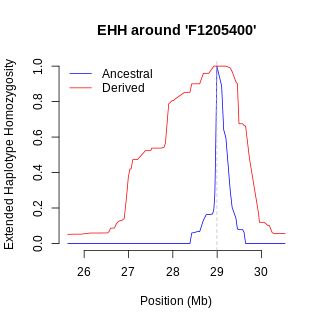For comparison, we might ignore that haplotypes have been phased and set the option phased to FALSE. Figure \@ref(fig:ehh2) shows that for this particular marker the differences are rather small:

plot(calc_ehh(haplohh_cgu_bta12,
mrk = "F1205400",
phased = FALSE),
xlim = c(2.55E7, 3.05E7))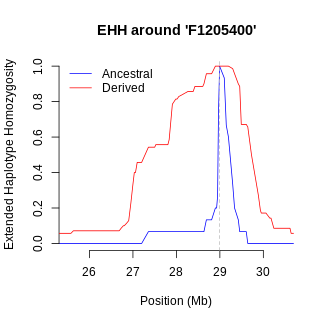## The function calc_ehhs()

The calc_ehhs() function computes $$\mathrm{EHHS}$$ and $$\mathrm{nEHHS}$$ around the focal marker $$s$$ relative to another marker $$t$$. This function also computes the corresponding integrals $$\mathrm{iES}$$ and $$\mathrm{inES}$$ respectively. The options are identical to those of the function calc_ehh (see previous section), except that polarized is not needed here. Details are available by the command:

?calc_ehhs


In the following example, the EHHS statistics are computed around the SNP F1205400.

data(haplohh_cgu_bta12)
res <- calc_ehhs(haplohh_cgu_bta12,
mrk = "F1205400",
include_nhaplo = TRUE)


The output is similar to that of calc_ehh except that there are no alleles to be distinguished, but instead the normalized and non-normalized versions of EHHS. A list with four elements is obtained:

1. mrk.name: the name/identifier of the focal marker.
2. ehhs: a data frame with EHHS and nEHHS values along the chromosome around the focal marker. Optionally, the column NHAPLO can be included to show how many chromosomes were evaluated at each marker.
3. IES: iES, i.e. the integral over the EHHS curve.
4. INES: inES, i.e. the integral over the nEHHS curve.
res$mrk.name  >  "F1205400"  res$ehhs

>          POSITION       EHHS      NEHHS NHAPLO
> F1204840 26132031 0.00000000 0.05132310    280
> F1204850 26189286 0.00000000 0.05132310    280
> F1204870 26255986 0.00000000 0.05132310    280
> F1204880 26313792 0.00000000 0.05136758    280
> F1204900 26479781 0.00000000 0.05167890    280
> F1204910 26507987 0.00000000 0.05167890    280
> F1204920 26526646 0.00000000 0.05167890    280
> F1204930 26558947 0.00000000 0.05777185    280
> F1204940 26599290 0.00000000 0.07480543    280
> F1204950 26683271 0.00000000 0.07480543    280
> F1204960 26727974 0.05499232 0.09553035    280
> F1204970 26779948 0.06190476 0.10753836    280
> F1204980 26800201 0.06318484 0.10976206    280
> F1204990 26854850 0.06464414 0.11229709    280
> F1205000 26902773 0.06899642 0.11985768    280
> F1205010 26952729 0.12450077 0.21627752    280
> F1205020 26997449 0.18312852 0.31812319    280
> F1205030 27031612 0.20486431 0.35588170    280
> F1205040 27058533 0.20509473 0.35628197    280
> F1205050 27097980 0.23049155 0.40040027    280
> F1205070 27200291 0.23049155 0.40040027    280
> F1205080 27356111 0.25163850 0.43713587    280
> F1205090 27378189 0.25517153 0.44327329    280
> F1205100 27465862 0.25517153 0.44327329    280
> F1205110 27501592 0.25519713 0.44331777    280
> F1205120 27525360 0.26231439 0.45568157    280
> F1205130 27588853 0.26233999 0.45572604    280
> F1205150 27735666 0.26233999 0.45572604    280
> F1205160 27804138 0.26490015 0.46017345    280
> F1205170 27833642 0.27667691 0.48063153    280
> F1205180 27907564 0.38374296 0.66662219    280
> F1205190 27985596 0.39272913 0.68223260    280
> F1205200 28014498 0.39298515 0.68267734    280
> F1205210 28054852 0.39782386 0.69108294    280
> F1205230 28266218 0.41610343 0.72283745    280
> F1205240 28296968 0.41610343 0.72283745    280
> F1205250 28386447 0.41610343 0.72283745    280
> F1205260 28426219 0.44129544 0.76659996    280
> F1205270 28562210 0.44206349 0.76793418    280
> F1205280 28606777 0.44211470 0.76802313    280
> F1205290 28633388 0.45343062 0.78768068    280
> F1205300 28689805 0.47662570 0.82797421    280
> F1205310 28721292 0.47800819 0.83037581    280
> F1205320 28753422 0.47946749 0.83291083    280
> F1205330 28786001 0.47946749 0.83291083    280
> F1205340 28810078 0.47946749 0.83291083    280
> F1205360 28896769 0.49447005 0.85897265    280
> F1205370 28925117 0.50171531 0.87155882    280
> F1205380 28947722 0.50952381 0.88512342    280
> F1205390 28967990 0.53479263 0.92901935    280
> F1205400 28993983 0.57565284 1.00000000    280
> F1205420 29101326 0.56541219 0.98221036    280
> F1205440 29147373 0.54295955 0.94320658    280
> F1205450 29197279 0.53868408 0.93577941    280
> F1205460 29295437 0.50642601 0.87974205    280
> F1205470 29337933 0.48806964 0.84785412    280
> F1205480 29424210 0.45381464 0.78834779    280
> F1205490 29456634 0.44365079 0.77069157    280
> F1205500 29492629 0.33402458 0.58025350    280
> F1205530 29576146 0.33402458 0.58025350    280
> F1205540 29607285 0.32793139 0.56966867    280
> F1205550 29642190 0.32501280 0.56459862    280
> F1205560 29727300 0.23663594 0.41107405    280
> F1205570 29885809 0.12012289 0.20867245    280
> F1205580 29933214 0.08553507 0.14858795    280
> F1205590 29953532 0.06075269 0.10553702    280
> F1205600 30060316 0.06075269 0.10553702    280
> F1205610 30148780 0.05192012 0.09019346    280
> F1205620 30176268 0.05192012 0.09019346    280
> F1205640 30241555 0.00000000 0.05532577    280
> F1205650 30270049 0.00000000 0.05154547    280
> F1205660 30472702 0.00000000 0.05012230    280
> F1205680 30532042 0.00000000 0.05012230    280

res$IES  >  936407.6  res$INES

>  1760565


Figure \@ref(fig:ehhs) can be obtained by setting the output of calc_ehhs into the function plot.ehhs():

plot(res)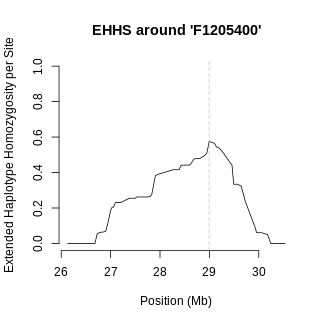By default, the un-normalized EHHS values are plotted. This can be toggled by setting nehhs = TRUE. As can be seen in Figure \@ref(fig:nehhs), the values are scaled to yield 1 at the focal marker.

plot(res, nehhs = TRUE)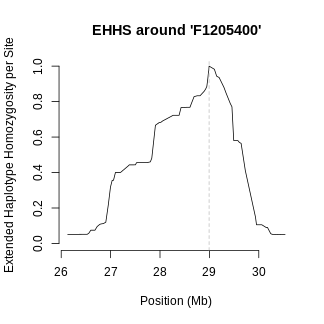The parameter phased of the function calc_ehhs() can be toggled to FALSE yielding Figure \@ref(fig:ehhs2). As described above, for unphased data the EHHS values are always normalized. For this particular marker we get a similar picture as for phased nEHHS.

plot(calc_ehhs(haplohh_cgu_bta12,
mrk = "F1205400",
phased = FALSE))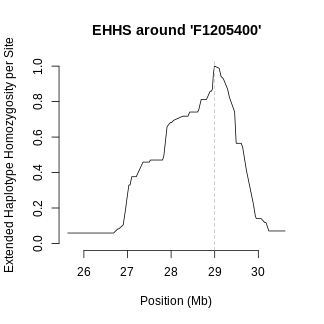## The function scan_hh() {#scanhh}

While the functions calc_ehh() and calc_ehhs() return the integrated statistics iHH and iES for a particular marker, the function scan_hh() calculates these values for all markers in the haplohh object. However, in contrast to calc_ehh() which computes an iHH value for each allele of a possibly multi-allelic focal marker, scan_hh() calculates only two iHH values, namely (by default) for the ancestral allele and the derived allele with highest frequency. In case of unpolarized markers (see section \@ref(polarizing)), the option polarized should be set to FALSE, causing the function to use major and minor (most frequent and second most frequent) alleles instead.

The remainder of options are essentially the same as for the functions calc_ehh() and calc_ehhs() (see section \@ref(EHH)). Details are given in the documentation:

?scan_hh


As an example, in order to scan the haplohh_cgu_bta12 object (containing data on 1424 SNPs for 280 haplotypes), one may use the following command:

data(haplohh_cgu_bta12)
scan <- scan_hh(haplohh_cgu_bta12)


The resulting object scan is a data frame with 1424 rows (the number of markers declared in the haplohh object) and 10 columns:

1. chromosome name
2. position in the chromosome
3. sample frequency of the ancestral / major allele
4. sample frequency of the second-most frequent remaining allele
5. number of evaluated haplotypes at the focal marker for the ancestral / major allele
6. number of evaluated haplotypes at the focal marker for the second-most frequent remaining allele
7. iHH of the ancestral / major allele
8. iHH of the second-most frequent remaining allele
9. iES
10. inES

Note that while for phased data the number of evaluated haplotypes of a core allele corresponds to its frequency in the sample, in case of unphased data the evaluation is restricted to haplotypes of homozygous individuals.

The following command prints a chunk of the scan containing the marker F1205400:

scan[453:459,]

>          CHR POSITION     FREQ_A    FREQ_D NHAPLO_A NHAPLO_D     IHH_A
> F1205370  12 28925117 0.06071429 0.9392857       17      263  765720.1
> F1205380  12 28947722 0.82500000 0.1750000      231       49 1477031.7
> F1205390  12 28967990 0.90000000 0.1000000      252       28 1211909.5
> F1205400  12 28993983 0.30357143 0.6964286       85      195  284429.9
> F1205420  12 29101326 0.01785714 0.9821429        5      275  269322.2
> F1205440  12 29147373 0.05714286 0.9428571       16      264  336600.7
> F1205450  12 29197279 0.04285714 0.9571429       12      268  528732.6
>              IHH_D       IES    INES
> F1205370 1121814.8  971728.6 1119699
> F1205380  454891.9  966416.3 1433143
> F1205390  574301.6  954903.3 1204234
> F1205400 2057107.4  936407.6 1760565
> F1205420 1086530.3 1041943.5 1086279
> F1205440 1185898.6 1036274.2 1182946
> F1205450 1149951.0 1039850.3 1148696


Note that scan_hh() is more efficient than calc_ehh() and calc_ehhs() applied consecutively for each marker as can be seen by running the two code snippets below:

# perform scan using scan_hh
system.time(scan <- scan_hh(haplohh_cgu_bta12))

>    user  system elapsed
>   0.862   0.001   0.862

# perform scan applying calc_ehh and calc_ehhs to each marker
slow_scan_hh <- function(haplohh) {
# create empty vectors of size nmrk
IHH_A <- IHH_D <- IES <- INES <- vector("numeric", nmrk(haplohh))
# invoke calc_ehh and calc_ehhs for each marker
for (i in 1:nmrk(haplohh)) {
res <- calc_ehh(haplohh, mrk = i)
IHH_A[i] <- res$ihh["IHH_A"] IHH_D[i] <- res$ihh["IHH_D"]
res <- calc_ehhs(haplohh, mrk = i)
IES[i] <- res$IES INES[i] <- res$INES
}
# create data frame (the return value of this function)
data.frame(IHH_A = IHH_A,
IHH_D = IHH_D,
IES = IES,
INES = INES)
}
system.time(slow_scan <- slow_scan_hh(haplohh_cgu_bta12))

>    user  system elapsed
>   4.867   0.004   4.870


Comparing columns shows that the computed values are identical:

identical(slow_scan[, "IHH_A"], scan[, "IHH_A"])

>  TRUE

identical(slow_scan[, "IHH_D"], scan[, "IHH_D"])

>  TRUE

identical(slow_scan[, "IES"], scan[, "IES"])

>  TRUE

identical(slow_scan[, "INES"], scan[, "INES"])

>  TRUE


# Computing iHS, Rsb and XP-EHH

## The iHS within-population statistic

### Definition {#ihs}

The abbreviation iHS refers to “integrated haplotype homozygosity score”. Let uniHS represent the un-standardized log-ratio of ancestral iHH$$^A$$ to derived iHH$$^D$$ of a certain focal marker $$s$$: $\mathrm{uniHS}(s)=\ln\left(\frac{\mathrm{iHH}^A(s)}{\mathrm{iHH}^D(s)}\right)$ Following [@Voight2006] we perform a standardization by setting: $\mathrm{iHS}(s)=\frac{\mathrm{uniHS}(s) - \mathrm{mean}(\mathrm{uniHS}|p_s)}{\mathrm{sd}(\mathrm{uniHS}|p_s)}$ where $$\mathrm{mean}(\mathrm{uniHS}|p_s)$$ and $$\mathrm{sd}(\mathrm{uniHS}|p_s)$$ represent the mean and standard deviation of uniHS restricted to those markers with a similar derived allele frequency as observed at the focal marker ($$p_s$$). In practice, the markers are binned so that each bin contains not a single frequency but a small range of frequencies to yield enough markers for reliable estimates of mean and standard deviation.

iHS is constructed to have an approximately standard Gaussian distribution and to be comparable across markers regardless of their underlying allele frequencies.

For a practical characterization of “outliers” we stretch statistical notation and associate a p-value to iHS [@Gautier2011] by: $p_\mathrm{iHS}=-\log_{10}\left(2\Phi\left(-|\mathrm{iHS}|\right)\right)$ where $$\Phi\left(x\right)$$ represents the Gaussian cumulative distribution function.

Assuming most of genotyped markers behave neutrally and the distribution of iHS values for neutral markers follows indeed a standard Gaussian distribution, we might interpret $$p_\text{iHS}$$ as a two-sided p-value (on a $$-\log_{10}$$ scale) of a test on the null hypothesis of no selection.

Alternatively, a one-sided p-value (in a $$-\log_{10}$$ scale) might be defined [@Gautier2011] as: \begin{equation} p\text{left}\text{iHS}=-\log{10}\left(\Phi\left(\text{iHS}\right)\right) \end{equation} allowing the identification of those sites where the derived allele displays a significantly higher extended haplotype homozygosity than the ancestral allele or \begin{equation} p\text{right}\text{iHS}=-\log{10}\left(1-\Phi\left(\text{iHS}\right)\right) \end{equation} for the opposite case.

In case of unpolarized alleles, the iHH values of major and minor alleles are opposed to obtain uniHS. Since derived allele frequency cannot be accounted for, no binning should be performed. The resulting standardized iHS cannot be expected to follow a normal distribution and p-values are not meaningful.

### The function ihh2ihs() {#ihh2ihs}

The ihh2ihs() function computes iHS using a data frame containing the iHH values for ancestral and derived (resp. major and minor) alleles as obtained by the scan_hh() function (see section \@ref(scanhh)). The argument min_maf allows to exclude focal markers according to their minor allele frequency (by default min_maf=0.05). The argument freqbin controls the size (or number) of the allele frequency bins used to perform standardization (see section \@ref(ihs)). More precisely, allele frequency bins are built from min_maf to 1-min_maf in steps of size freqbin (by default freqbin=0.025). If an integer of 1 or greater is specified, a corresponding number of equally spaced bins is created. If freqbin is set to 0, standardization is performed considering each observed frequency as a discrete frequency class, which is useful when there are only a few distinct haplotypes.

For unphased data, iHH is calculated using only haplotypes of individuals which are homozygous at the focal marker. This number can be considerably lower than the absolute allele frequency. Hence, in addition to min_maf, the option min_nhaplo (default NA) should be used to reduce statistical noise arising from too few evaluated haplotypes.

Optionally, the allele frequencies of the input data frame can be included into the output data frame by setting include_freq to TRUE.

A p-value is calculated for standardized iHS values. By default, it is two-sided, but a side can be chosen by setting argument p.side to "left" or "right".

As a typical workflow for performing a whole genome scan one might run scan_hh() on haplotype data from each chromosome and concatenate the resulting data frames before standardization. In the following example, we assume that the haplotype files are named as hap_chr_i.cgu with $$i=1,…,29$$ and the marker information file is named map.inp. The data frame wgscan contains the $$iHH^A$$ and $$iHH^D$$ values for the whole genome and serves as input for the ihh$ihs() function which calculates iHS, i.e. the standardized log ratio of the two $$iHH$$ values, for each marker. ## demo code - no data files for all chromosomes provided for(i in 1:29) { # haplotype file name for each chromosome hap_file = paste("hap_chr_", i, ".cgu", sep = "") # create internal representation hh <- data2haplohh(hap_file = hap_file, map_file = "map.inp", chr.name = i, allele_coding = "map") # perform scan on a single chromosome (calculate iHH values) scan <- scan_hh(hh) # concatenate chromosome-wise data frames to # a data frame for the whole genome # (more efficient ways certainly exist...) if (i == 1) { wgscan <- scan } else { wgscan <- rbind(wgscan, scan) } } # calculate genome-wide iHS values wgscan.ihs <- ihh2ihs(wgscan)  For illustration, the object wgscan.cgu contains the calculated and concatenated $$iHH$$ values of a whole genome scan on population CGU [@Gautier2011]: library(rehh.data) data(wgscan.cgu) wgscan.ihs.cgu <- ihh2ihs(wgscan.cgu)  > Discard focal markers with Minor Allele Frequency equal to or below 0.05 . > 6890 markers discarded. > 37167 markers remaining.  The resulting object wgscan.ihs.cgu is a list with two elements: 1. ihs: a data frame with iHS and corresponding p-value $$p_\mathrm{iHS}$$ (p-value in a $$-\log_{10}$$ scale assuming the iHS are normally distributed under the neutral hypothesis). The first rows are displayed below: head(wgscan.ihs.cgu$ihs)

>          CHR POSITION        IHS LOGPVALUE
> F0100190   1   113642 -0.5582992 0.2390952
> F0100220   1   244699  0.2723337 0.1049282
> F0100250   1   369419  0.4810736 0.2003396
> F0100270   1   447278  1.0618710 0.5401640
> F0100280   1   487654  0.8184060 0.3839181
> F0100290   1   524507 -0.3897024 0.1569189

1. frequency.class: a data frame summarizing the derived allele frequency bins used for standardization and mean and standard deviation of the un-standardized values. The first rows are shown below:
head(wgscan.ihs.cgu$frequency.class)  > N_MRK MEAN_UNIHS SD_UNIHS LOWER_QT UPPER_QT > [0.05,0.075) 1090 -0.7117920 0.6106032 -2.030274 0.2952986 > [0.075,0.1) 907 -0.5788939 0.5703576 -1.833828 0.3448781 > [0.1,0.125) 787 -0.4572926 0.4875843 -1.563436 0.3611656 > [0.125,0.15) 837 -0.3992265 0.4542230 -1.403821 0.3629034 > [0.15,0.175) 824 -0.3484786 0.4341126 -1.253109 0.4850874 > [0.175,0.2) 886 -0.2808167 0.4621221 -1.297452 0.5788857  ## The Rsb pairwise population statistic ### Definition {#rsb} The abbreviation Rsb stands for “ratio of EHHS between populations”. For a given marker $$s$$, let $\mathrm{unRsb}(s)=\ln\left(\frac{\mathrm{inES}_\text{pop1}(s)}{\mathrm{inES}_\text{pop2}(s)}\right)$ represent the log-ratio of the inES values computed in two populations $$pop1$$ and $$pop2$$ (see section \@ref(iES)). The Rsb for marker $$s$$ is defined as the standardized unRsb [@Tang2007]: \begin{equation} \mathrm{Rsb(s)}=\frac{\mathrm{unRsb}(s) - \text{median}({\mathrm{unRsb}})}{\mathrm {sd}({\mathrm{unRsb})}} \end{equation} where $$\text{median}(\mathrm{unRsb})$$ and $$\mathrm{sd}(\mathrm{unRsb})$$ represent the median and standard deviation of unRsb computed over all analyzed markers. Note that for the sake of uniformity, we included the logarithm into the definition of the quantity while in the original article it remained outside. However, we follow [@Tang2007] in using the median instead of the mean (hence unlike the definitions of iHS and XP-EHH). The authors argue that this might increase the robustness against different demographic scenarios. Like XP-EHH, but unlike $$iHS$$, no binning with respect to allele frequencies is performed (see section \@ref(polarizing)). As iHS (see section \@ref(ihs)), Rsb is constructed to have an approximately standard Gaussian distribution and may be transformed analogously into a p-value $$p_\text{Rsb}$$ (in a $$-\log_{10}$$ scale), either two-sided or one-sided. As for the latter, $$p^\text{left}_\text{Rsb}$$ identifies strong extended homozygosity in population $$pop2$$ relative to population $$pop1$$ and vice versa for $$p^\text{right}_\text{Rsb}$$. ### The function ines2rsb() {#ines2rsb} The ines2rsb() function computes Rsb using two data frames containing each the inES statistics of one population as obtained by the scan_hh() function (see section \@ref(scanhh)). As with iHS the inES values have to be calculated chromosome-wise and concatenated afterwords (for each population separately) like in the example of section \@ref(ihh2ihs). The Rsb values are computed for all markers present in both data frames. They are identified by chromosome name and position, not their identifiers, thus within each population the chromosomal positions must be unique. Optionally, the allele frequencies of both populations can be taken over from the input data frames (if present there) to the output data frame by setting include_freq to TRUE. For illustration, we take calculated and concatenated $$inES$$ values from a genome scan [@Gautier2011] provided as example data and compute for each SNP the Rsb between the CGU and EUT populations: data(wgscan.cgu) ; data(wgscan.eut) ## results from a genome scan (44,057 SNPs) see ?wgscan.eut and ?wgscan.cgu for details rsb.cgu_eut <- ines2rsb(scan_pop1 = wgscan.cgu, scan_pop2 = wgscan.eut, popname1 = "CGU", popname2 = "EUT")  > Scan of pop1 contains 44057 markers. > Scan of pop2 contains 44057 markers. > Merged data contains 44057 markers.  The resulting object rsb.cgu_eut is a data frame which shows for each marker its Rsb and associated p-value. The latter are by default two-sided, but a side can be chosen by setting argument p.side to "left" or "right". The first rows of the resulting data frame are printed below: head(rsb.cgu_eut)  > CHR POSITION RSB_CGU_EUT LOGPVALUE > F0100190 1 113642 -0.3398574 0.13432529 > F0100220 1 244699 -1.0566283 0.53658299 > F0100250 1 369419 -0.1468326 0.05390941 > F0100270 1 447278 -1.8191608 1.16186336 > F0100280 1 487654 -0.2193069 0.08280392 > F0100290 1 524507 -0.7941300 0.36945032  ## The XP-EHH pairwise population statistic ### Definition The XP-EHH (cross-population EHH) statistic [@Sabeti2007] is similar to Rsb except that it is based on iES instead of inES (see section \@ref(iES)). Hence, for or a given marker $$s$$, let $\text{unXP-EHH}(s)=\ln\left(\frac{\mathrm{iES}_\text{pop1}(s)}{\mathrm{iES}_\text{pop2}(s)}\right)$ represent the log-ratio of the iES values computed by the function scan_hh() in the populations $$pop1$$ and $$pop2$$ (see section \@ref(iES)). The XP-EHH for a given focal marker is defined as the standardized unXP-EHH [@Sabeti2007]: \begin{equation} \text{XP-EHH}(s)=\frac{\text{unXP-EHH}(s) - \text{mean}(\text{unXP-EHH})}{\text{sd}(\text{unXP-EHH})} \end{equation} where $$\text{mean}(\text{unXP-EHH})$$ and $$\text{sd}(\text{unXP-EHH})$$ represent the mean and standard deviation of unXP-EHH computed over all analyzed markers. As with Rsb, the information about the ancestral and derived status of alleles at the focal marker does not figure in the formula and no binning is performed. As with the previous two scores iHS (section \@ref(ihs)) and Rsb (section \@ref(rsb)), XP-EHH is constructed to have an approximately standard Gaussian distribution and may be transformed analogously into a p-value $$p_\text{XP-EHH}$$ (in a $$-\log_{10}$$ scale), either two-sided or one-sided. Among the latter, $$p^\text{left}_\text{XP-EHH}$$ identifies strong extended homozygosity in population $$pop2$$ relative to population $$pop1$$ and vice versa for $$p^\text{right}_\text{XP-EHH}$$. ### The function ies2xpehh() {#ies2xpehh} The ies2xpehh() function computes XP-EHH using two data frames, one for each population, containing the iES statistics as obtained by the scan_hh() function (see section \@ref(scanhh)). As with iHS and Rsb, the iES have to be calculated chromosome-wise and concatenated afterwords (for each population separately) as has been shown in section \@ref(ihh2ihs). The XP-EHH values are computed for all markers present in both data frames. They are identified by chromosome name and position, not their identifiers, thus within each population the chromosomal positions must be unique. Optionally, the allele frequencies in both populations can be taken over from the input data frames (if present there) to the output data frame by setting include_freq to TRUE. For illustration, we take calculated and concatenated $$iES$$ values from a genome scan [@Gautier2011] and compute for each SNP the XP-EHH between the CGU and EUT populations: data(wgscan.cgu) ; data(wgscan.eut) ## results from a genome scan (44,057 SNPs) see ?wgscan.eut and ?wgscan.cgu for details xpehh.cgu_eut <- ies2xpehh(scan_pop1 = wgscan.cgu, scan_pop2 = wgscan.eut, popname1 = "CGU", popname2 = "EUT")  > Scan of pop1 contains 44057 markers. > Scan of pop2 contains 44057 markers. > Merged data contains 44057 markers.  The resulting object xpehh.cgu_eut is a data frame containing for each SNP the XP-EHH and associated p-value. The latter are by default two-sided, but a side can be chosen by setting argument p.side to "left" or "right". The first rows are displayed below: head(xpehh.cgu_eut)  > CHR POSITION XPEHH_CGU_EUT LOGPVALUE > F0100190 1 113642 -0.5943673 0.2578513 > F0100220 1 244699 -0.7903997 0.3672448 > F0100250 1 369419 -0.9273568 0.4513142 > F0100270 1 447278 -0.3858354 0.1551387 > F0100280 1 487654 -0.9570604 0.4703941 > F0100290 1 524507 -0.7908863 0.3675322  ## Delineating regions with “outliers”: the function calc_candidate_regions() In contrast to neutral evolution, selection tends to produce clusters of markers with outlier values [@Tang2007]. For this reason, typically not (only) the markers with outlier scores are reported, but intervals with a conspicuous number or percentage of such markers. Although often similar ad-hoc procedures can be found in the literature, their respective parameters vary widely. We offer the function calc_candidate_regions() which scans through the genome in (possibly overlapping) sliding windows and identifies those as “candidates” which fulfill three conditions: • a minimum number of markers (min_n_mrk) • a minimum number of extremal markers (min_n_extr_mrk) • a minimum percentage of extremal markers among the markers (min_perc_extr_mrk). Markers with extreme values are those with a score greater than specified by parameter threshold. Apart from the values of the above conditions, the average of all marker scores and the average of extremal scores is reported for each candidate window. By default, neighboring and overlapping candidate windows are joined together and the associated values recalculated. This can be turned off by setting join_neighbors to FALSE. If window_size is set to 1, only the set of extremal markers is returned. B default, the score itself is tested and reported. Toggling pval to TRUE, uses the associated p-value instead. cr.cgu <- calc_candidate_regions(wgscan.ihs.cgu, threshold = 4, pval = TRUE, window_size = 1E6, overlap = 1E5, min_n_extr_mrk = 2) cr.cgu  > CHR START END N_MRK MEAN_MRK MAX_MRK N_EXTR_MRK PERC_EXTR_MRK > 1 1 61300000 63200000 39 0.7998786 4.392531 3 7.69 > 2 4 48200000 50100000 33 1.0550337 5.993989 3 9.09 > 3 5 27500000 29400000 23 2.3879767 6.079756 3 13.04 > 4 5 79500000 81300000 28 0.8225111 6.752500 2 7.14 > 5 7 52100000 53200000 16 1.8344229 5.019538 2 12.50 > 6 12 28000000 29900000 22 2.6578336 6.294289 3 13.64 > 7 18 11600000 13500000 30 1.9630287 6.203345 3 10.00 > MEAN_EXTR_MRK > 1 4.332750 > 2 5.593718 > 3 4.779048 > 4 5.409110 > 5 4.705576 > 6 5.276263 > 7 5.026240  \clearpage # Visualization of the statistics ## Un-standardized iHS: the function freqbinplot() {#freqbinplot} Since mutations and recombinations tend to shorten stretches of homozygosity between chromosomes, an ancestral (older) allele is expected to have lower extended haplotype homozygosity than a derived (younger) allele. Hence the distribution of unstandardized $$iHS=\ln(\frac{iHH^A}{iHH^D})$$ is biased towards negative values. More importantly, there is a additional dependency on both ancestral and derived allele frequencies (in the bi-allelic case one is determined by the other). This can be seen by setting the output of the function ihh2ihs into the function freqbinplot() yielding Figure \@ref(fig:freqbin). freqbinplot(wgscan.ihs.cgu)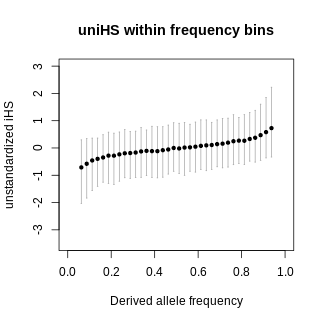Note that this frequency dependence is expected under neutral evolution and not due to selection. In fact, an implicit assumption of the bin-wise standardization is that each bin is dominated by neutral markers. Thus, the bin-wise standardization reduces the dependency of iHS on the allele frequency; see section \@ref(polarizing) of this vignette and Figure 4 in [@Voight2006] [In their case the effect is even more pronounced, because they polarized alleles with help of an outgroup species while in the case of our data it was done using extant ancestral populations as a proxy.] ## Rsb vs. XP-EHH comparison Figure \@ref(fig:comp) shows a plot of Rsb against XP-EHH values across the CGU and EUT populations (see section \@ref(ines2rsb) and \@ref(ies2xpehh) respectively). Marked in red is the marker that was repeatedly used in the examples above. The plot was generated using the following R code: plot(rsb.cgu_eut[, "RSB_CGU_EUT"], xpehh.cgu_eut[, "XPEHH_CGU_EUT"], xlab = "Rsb", ylab = "XP-EHH", pch = ".", xlim = c(-7.5, 7.5), ylim = c(-7.5, 7.5)) # add red circle for marker with name "F1205400" points(rsb.cgu_eut["F1205400", "RSB_CGU_EUT"], xpehh.cgu_eut["F1205400", "XPEHH_CGU_EUT"], col = "red") # add dashed diagonal abline(a = 0, b = 1, lty = 2)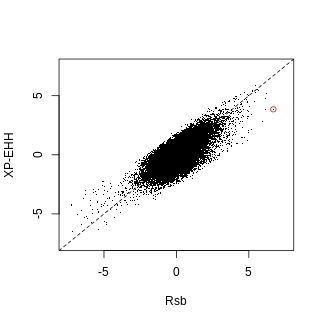## Distribution of standardized values: the function distribplot() The distribplot() function allows to visualize the distributions of the standardized scores (either iHS, Rsb or XP-EHH) and compare them to the standard Gaussian distribution. As an example, in Figure \@ref(fig:distribplot) the function was applied on the iHS estimates obtained for the CGU population (see section \@ref(ihs)): distribplot(wgscan.ihs.cgu$ihs$IHS, xlab = "iHS")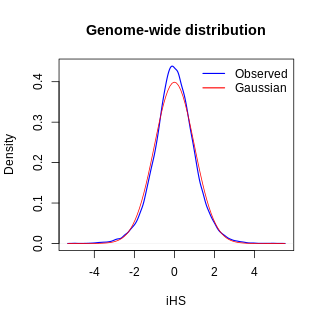Setting the option qqplot to TRUE toggles the output to a qqplot, as in Figure \@ref(fig:qqplot). distribplot(wgscan.ihs.cgu$ihs$IHS, xlab = "iHS", qqplot = TRUE)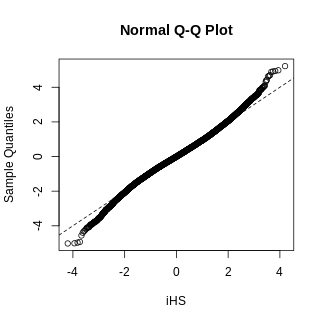## Genome wide score plots: the function manhattanplot() The function manhattanplot() draws a Manhattan plot of the whole genome scores obtained by the functions ihh2ihs(), ines2rsb() or ies2xpehh(). Figure \@ref(fig:manhattanplot) was drawn using the command: manhattanplot(wgscan.ihs.cgu, main = "iHS (CGU cattle breed)")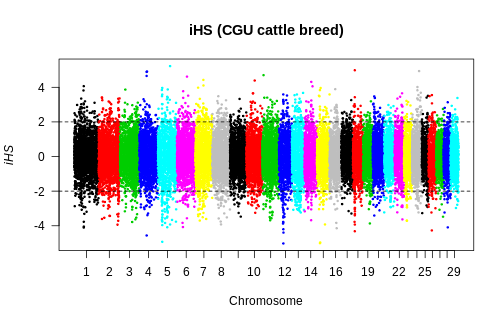If the option pval is switched to TRUE the associated p-value instead of the score itself is plotted (Figure \@ref(fig:pval)). manhattanplot(wgscan.ihs.cgu, pval = TRUE, threshold = 4, main = "p-value of iHS (CGU cattle breed)")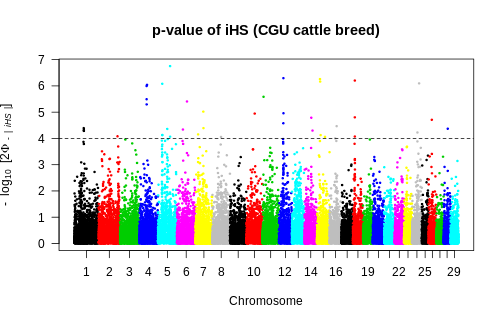The colors of the chromosomes in Figures \@ref(fig:manhattanplot) and \@ref(fig:pval) are the default colors of R as obtained by the command palette(). It is possible to change them by the same command. Note that the colors are associated with the order of chromosomes in the scan and not their order in the plot. Candidate regions as obtained by the function calc_candidate_regions() can be added to the plot as parameter cr. Individual markers can be highlighted by setting argument mrk to a vector of marker IDs or a data frame with positions (containing columns with name CHR and POSITION). In order to reduce the number of plotted data points, the data set can be rasterized in both dimensions by parameter resolution. The data points are then rounded to the specified resolution and duplicate points removed. Furthermore, it is possible to specify a subset or a re-ordering of chromosomes with help of parameter chr.name as in Figure \@ref(fig:manhattanplotsub). # re-define colors palette(c("red", "green")) manhattanplot(wgscan.ihs.cgu, pval = TRUE, threshold = 4, chr.name = c("1", "4", "5", "12"), main = "iHS (CGU cattle breed)", cr = cr.cgu, mrk = "F1205400", resolution = c(200000, 0.05))  > Rasterization reduced 6958 data points to 6377 .  # set back to default colors palette("default")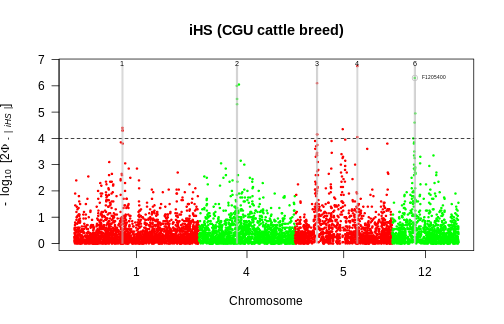## Genome wide score plots: the function manhattan() of package qqman The package qqman contains a function manhattan() which is similar to the function manhattanplot() of this package. The input data frame is expected to have a slightly different format, though. Hence, before plotting we need to “translate” our data as in the following example with ihs values: # extract data frame from result list ihs <- wgscan.ihs.cgu$ihs
# create new data frame
wgscan.cgu.ihs.qqman <- data.frame(
CHR = as.integer(factor(ihs$CHR, levels = unique(ihs$CHR))),
# chromosomes as integers
BP = ihs$POSITION, # base pairs P = 10**(-ihs$LOGPVALUE),  # transform back to p-values
SNP = row.names(ihs)       # SNP names
)


We can now use the new data frame as input for the function manhattan() to obtain Figure \@ref(fig:qqman):

library(qqman)
manhattan(wgscan.cgu.ihs.qqman,
col = c("red","green"),
chrlabs = unique(ihs$CHR), suggestiveline = 4, highlight = "F1205400", annotatePval = 0.0001)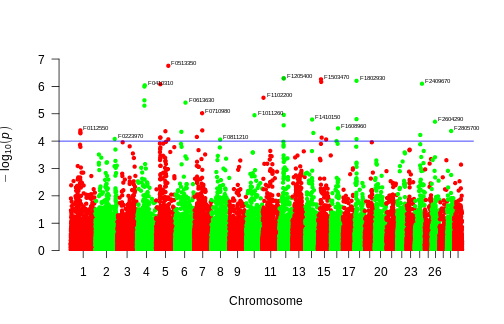\clearpage # Visualization of the haplotype structure ## The function plot.haplohh() Chunks of the haplotype matrix can be plotted, provided they are not too large, with sequences in rows and markers in columns. The following plot shows a chunk of 200 SNPs in the vicinity of SNP F1205400 which is identified by the dashed line. The derived alleles are marked in red, the ancestral ones not shown. hh_subset <- subset(haplohh_cgu_bta12, select.mrk = 350:550) oldpar <- par(mar = c(3, 2, 2, 2) + 0.1) plot( hh_subset, mrk = "F1205400", group_by_allele = TRUE, ignore.distance = TRUE, col = c(NA, "red"), linecol = c("lightblue", "lightpink"), mrk.col = "black", cex = 0.1, pos.lab.hap = "none", pos.lab.mrk = "none" ) par(oldpar)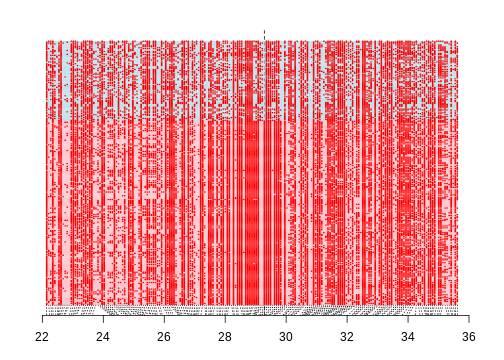The sequences are ordered with respect to the alleles of SNP F1205400: the lower two thirds of sequences contain the derived allele and are more homogenous around the SNP than the sequences carrying the ancestral allele. \clearpage ## The functions calc_furcation() and plot.furcation() Furcation structures portray the decay of haplotype homozygosity around a focal marker [@Sabeti2002]. They represent the complete information contained in the concept of extended shared haplotypes of which EHH and EHHS can be regarded as summary statistics. For each allele of a focal marker and each side (“left” or “right”), furcations arise when the extension step reaches a marker whose alleles distinguish hitherto identical haplotypes. In case of bi-allelic markers without missing values these can be only bifurcations. The furcation structure for a specific allele and a specific side is hence a tree with its root at the focal marker. A stark contrast between furcation structures of different core alleles should correspond to outlier values of iHS. The function calc_furcation() calculates such a furcation structure around a focal marker. data(haplohh_cgu_bta12) furcation <- calc_furcation(haplohh_cgu_bta12, mrk = "F1205400")  The result is an object of class furcation, comprising a set of tree structures with some additional information, see ?"furcation-class" for technical details. The function plot.furcation() renders graphically the furcation object. Within the plot, the root (focal marker) is identified by a vertical dashed line. The diagram is bi-directional, portraying decay along both sides of the focal marker. The thickness of the lines corresponds to the number of chromosomes sharing a haplotype. As an illustration, Figure \@ref(fig:furc) shows the bifurcation diagrams for derived and ancestral allele of the marker F1205400. plot(furcation)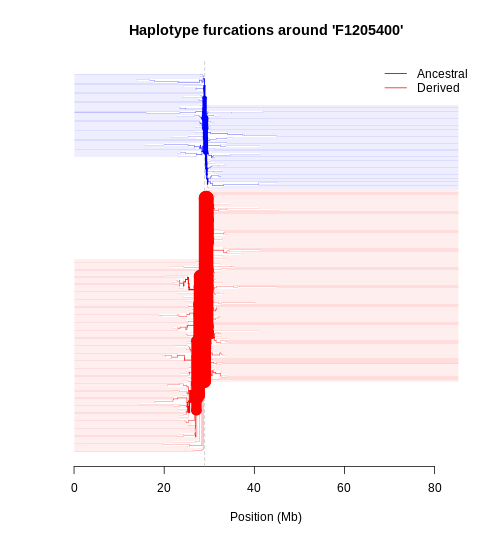It is possible to zoom into furcations using parameter xlim and label the leaves using the parameter hap.names, as shown in Figure \@ref(fig:furczoom), produced by the following command. Labels in bold indicate that further chromosomes are associated with the corresponding branch. plot(furcation, xlim = c(2.8E+7, 3E+7), lwd = 0.05, hap.names = hap.names(haplohh_cgu_bta12), cex.lab = 0.3)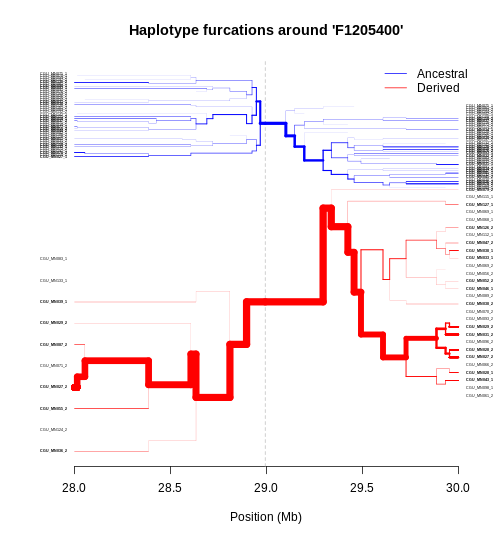Individual trees can be exported into Newick format. The function as.newick produces a (possibly very long) string in this format. The following command extracts the furcation tree of the ancestral allele on the left side of the marker: newick <- as.newick(furcation, allele = 0, side = "left", hap.names = hap.names(haplohh_cgu_bta12))  Such a string can be rendered graphically e.g. by the R package ape yielding Figure \@ref(fig:newick): library(ape) tree <- read.tree(text = newick) plot(tree, cex = 0.5, direction = "leftwards", edge.color = "blue", underscore = TRUE, no.margin = TRUE)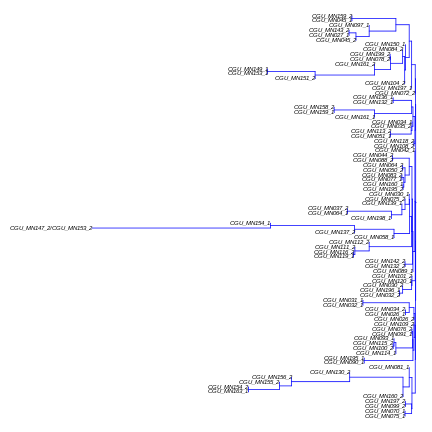## The functions calc_haplen() and plot.haplen() The length of a particular extended shared haplotype in a sample can be defined as the range that a chromosome shares a haplotype with at least one other chromosome. For a given chromosome it corresponds to the maximal extension of the inner branches to both sides of the focal marker in a furcation diagram. The function calc_haplen() calculates this quantity: haplen <- calc_haplen(furcation)  The haplen object is a list with four elements: 1. mrk.name: the name/identifier of the focal marker 2. position: the position of the focal marker 3. xlim: the position of first and last marker in the data set 4. haplen: a data frame with begin and end positions of the extended shared haplotypes. haplen$mrk.name

>  "F1205400"

haplen$position  >  28993983  haplen$xlim

>     79823 85277438

head(haplen$haplen)  > ALLELE DESCRIPTION MIN MAX > 1 0 Ancestral 28054852 29424210 > 2 0 Ancestral 28810078 30241555 > 3 0 Ancestral 23020095 31105214 > 4 1 Derived 25932431 31009388 > 5 1 Derived 24259278 32960675 > 6 1 Derived 26683271 31457115  Haplotype length is visualized in Figure \@ref(fig:haplo) obtained by the command plot(haplen)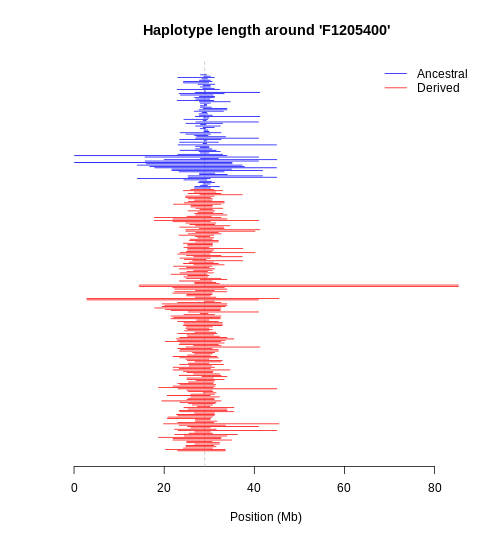As with a furcation plot, one can zoom into the picture using the parameter xlim and select alleles using parameter allele. The following command yields Figure \@ref(fig:haplozoom) showing only the region to the “left” of the focal marker and only the chromosomes of the ancestral core allele. plot(haplen, allele = 0, xlim = c(haplen$xlim, haplen\$position),
hap.names = hap.names(haplohh_cgu_bta12),
cex.lab = 0.3,
legend.xy.coords = "none")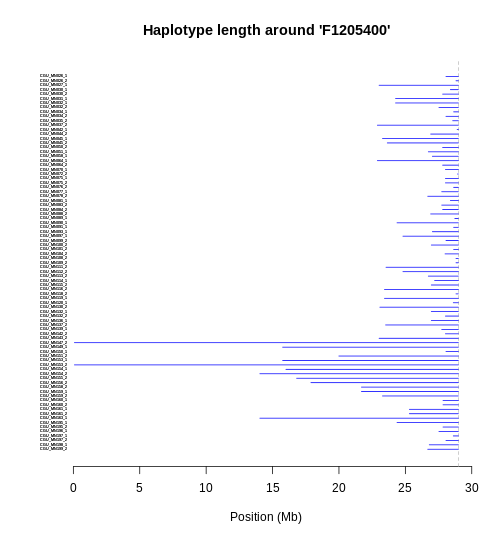We give a small example on how to check visual results by directly accessing the data of the haplohh-class object (see also its documentation by ?"haplohh-class"): from Figures \@ref(fig:newick) or \@ref(fig:haplozoom) it appears that two extended shared haplotypes reach the left border and we might identify them by their labels as CGU_MN147_2 and CGU_MN153_2. We can prove that there are indeed exactly two haplotypes in the sample which are identical within the complete region to the “left” of the focal marker:

# finding the index number of marker "F1205400"
mrk.nr = which(mrk.names(haplohh_cgu_bta12) == "F1205400")
# subset of all markers on the "left" of the focal one
hh_left = subset(haplohh_cgu_bta12, select.mrk = 1:mrk.nr)

> * Subset markers *
> 456 markers remaining.
> * Filtering data *
> Discard markers genotyped on less than 100 % of haplotypes.
> Data consists of 280 haplotypes and 456 markers.
> Number of mono-, bi-, multi-allelic markers:
> 1 2
> 6 450

# check for duplicated rows
which(duplicated(haplo(hh_left)))

> CGU_MN153_2
>         248

# row 248 is identical to a previous row, but which one?
# get the other row by a search in opposite direction
which(duplicated(haplo(hh_left), fromLast = TRUE))

> CGU_MN147_2
>         236

# extract the corresponding haplotype names
hap.names(hh_left)[c(236, 248)]

>  "CGU_MN147_2" "CGU_MN153_2"


Finally, after working through the vignette, we remove the example files from the current working directory:

remove.example.files()


\clearpage

# Data considerations

## Multi-allelic markers {#multiallelic}

For many species, a low per-site mutation rate ensures that the vast majority of Single Nucleotide Polymorphisms (SNPs) appears only with two alleles in a sample. Hence bi-allelic SNPs will constitute the foremost kind of data to apply our package onto. However, we think the ability to calculate the statistics for multi-allelic markers might be useful

• for species and/or genomic regions with a high per-site mutation rate
• for genetic variation in form of (short) tandem repeats or (larger) copy number variations which are multi-allelic by definition and may carry information not captured by SNP markers
• because the relative rarity of multi-allelic SNPs might make these particularly interesting
• since the original approach of [@Sabeti2002] was not to compare extended haplotype homozygosity between core alleles of a single SNP, but between multiple core haplotypes defined by several neighboring SNPs; such a partition could be created in rehh by adding an artificial multi-allelic marker.

## Dealing with gaps {#gaps}

Certain genomic regions such as centromeres are difficult to sequence and can give rise to large gaps between consecutive markers. If not accounted for, these will cause spuriously inflated values of the “integrals” iHH and iES. [@Voight2006] applied two corrections for gaps. First, they introduced a penalty proportional to physical distance that resulted in any gap being greater than 20 kb to be re-scaled to this number. Second, they discarded integration, if two consecutive markers with a distance greater than 200 kb were encountered. Both methods are implemented in rehh, yet turned off by default, since the corresponding thresholds should be adapted manually to the specific data set.

## Dealing with missing data {#missing}

Most phasing programs (such as fastPHASE or SHAPEIT2) interpolate (“impute”) missing genotypes, hence at least for phased SNP markers, missing values should be rather the exception than the rule.

In cases where missing values cannot be avoided, there is no generally accepted way of handling them. Some authors replace them probabilistically by the “background” frequency of alleles observed on the remainder chromosomes (i.e. a simple kind of “imputing”). We implemented instead the following approach: every time when the extension of shared haplotypes reaches a new marker, all chromosomes with missing values are removed from further calculations; exempt are chromosomes which at that point do not share any more a haplotype with another chromosome.

The number of chromosomes evaluated at each position is reported in the results of calc_ehh() resp. calc_ehhs(), if the parameter include_nhaplo is set to TRUE. If there are missing values, this number will decrease monotonically with increasing distance to the focal marker. Without missing values, the number will be constant until calculation is stopped, e.g. by reaching the boundary condition limehh(s).

Our approach to handle missing values has two non-obvious consequences: first, under certain circumstances, EHH or EHHS can transiently increase, namely if the removal of chromosomes leads accidentally to a more homogeneous set. Second, the normalization factor for nEHHS has to be recalculated based on the reduced set and is no longer constant over the entire region. For realistic data, however, both effects should be negligible.

## Dealing with multiple markers at the same position {#multiplemarkers}

Errors or typos aside, there are several possibilities how multiple markers with the same chromosomal position can arise:

• If positions are defined by genetic distances, two markers can have zero distance, if no recombination is observed between them.
• The variant call format allows to specify different kinds of markers in the same file. Hence at a certain position one might observe a SNP as well as an Insertion/Deletion or a tandem repeat.
• In output of ms the positions are given with a pre-set precision and consequently the positions of two different segregating sites might be rounded to the same number.

Since it is unclear how rehh should handle such markers, they are not accepted as input. Ideally, multiple markers should be dealt with by a pre-processing of the data outside of the package. As a quick-and-dirty work-around, we offer the option remove_multiple_markers, which, if set to TRUE, removes all but the first marker with identical positions.

## Dealing with unphased data {#phasing}

Notwithstanding expensive experimental methods, current high-throughput genotyping / sequencing technologies cannot directly assign alleles to specific chromosomes of a heterozygous diploid (or multiploid) individual. Instead, this task of phasing is typically performed by specialized bioinformatic tools like the previously mentioned SHAPEIT [@OConnell2014] and fastPHASE [@Scheet2006]. Although computationally demanding, the application of these tools is straight-forward and the results usually of sufficient quality for the calculation of EHH based statistics. Typically, the tools interpolate missing values away.

In the presumably rare cases where phasing is not feasible, EHH or EHHS can only be meaningfully estimated by reducing the set of compared chromosomes to those of homozygous (at the focal marker) individuals (assuming that the input data is ordered correspondingly). However, this reduction entails a substantial loss of power; even by an adapted parameter setting (see below) at the very minimum 10, but better up to 30 sequences are needed to obtain at least moderately accurate estimations.

For the within-population statistic iHS, the latter requirement concerns both major and minor alleles of a marker and scans should not be performed on samples comprising less than 100 sequences. Even for sample sizes of 200 sequences, meaningful estimation of iHS is hence restricted to variants of intermediate frequencies, i.e. high minor frequency.

For the cross-population statistics Rsb and XP-EHH a minimum number of 30 sequences from homozygous individuals is usually fulfilled if the sample size of each population exceeds 60 sequences.

For unphased sequences

• the option phased of the functions calc_ehh(), calc_ehhs() and scan_hh() must be set to FALSE. However, if the data is actually phased, this entails a substantial loss of power to detect selection!

Most of the variance (and hence “noise”) comes at any marker from the longest shared haplotypes. To limit their contribution

• the cut-off for the calculation of EHH resp. EHHS defined by option limehh resp. limehhs should be increased from the default value of 0.05 to 0.1.
• in function ihh2ihs(), hence for a within-population scan using iHH values, in addition to the filtering by the MAF of core alleles (parameter min_maf, default 0.05), a minimum absolute number of evaluated haplotypes should be set by parameter min_nhaplo; this value should never be lower than 10 and, if the sample size allows, be as high as 30.
• if the latter option is set to 20 or lower, a further cut-off, supplementing limehh, namely limhomohaplo should be set to 4, meaning that calculation of EHH/EHHS is stopped when less than 4 sequences (two individuals) remain homozygous.

See [@Klassmann2020] for a study on estimating iHS, Rsb and XP-EHH using unphased sequences.

## Dealing with unpolarized data {#polarizing}

The designation of alleles as 'ancestral' or 'derived' is referred to as polarization. Since sequences of ancient genomes are available only for a few species and restricted to a limited time back to the past, the 'ancestral' allele is usually inferred to be the one carried by one or more outgroup species such as chimpanzees or gorillas for humans. However, this presupposes the existence of a reference sequence of suitable 'neighbor' species of sufficient quality as well as reliable genome-wide alignments. These requirements are not trivial and even if they are fulfilled, any alignment will not cover the whole genome and the covered part will contain mis-specified alleles due to invisible secondary or back-mutations [@Baudry2003], possibly causing spurious signals of selection [@Hernandez2007].

Note that the bin-wise standardization of $$iHS$$ is the only calculation step within our package where the information about ancestry is exploited. The information of ancestry status is valuable since the expected values under neutral evolution depend on the respective allele frequencies at the focal marker (see Figure \@ref(fig:freqbin) of this vignette and Figure 4 of [@Voight2006]). The binning of markers by frequency before its standardization (see section \@ref(ihh2ihs)) is aimed to eliminate most of this dependence. For unpolarized alleles this correction cannot be done.

Hence two parameters are important when dealing with unpolarized data:

• since in this case the internal coding of alleles as 0,1,2 etc. conveys no information about ancestral status, it is appropriate to set the parameter polarized in function scan_hh to FALSE: iHH values are then computed for the major (most frequent) and minor (second-most frequent) alleles.
• the standardization of iHS for such data should NOT be done in a frequency-dependent manner and consequently the parameter freqbin in the function ihh2ihs() should be set to 1 (one bin).

Simulations have shown that neglecting ancestry status reduces the strength of the signal, yet conspicuous values remain clustered; consequently, a delineation of candidate regions of selection should rely primarily on this latter feature. For a more detailed analysis see [@Klassmann2020].

\clearpage

# Differences to the program hapbin {#hapbin}

The C++ program hapbin [@Maclean2015] is an alternative tool to calculate the statistics iHS and XP-EHH. The obtained values vary between hapbin and rehh. As far as we can tell, this is due to the differences in implementation listed below. The first 4 are hard-wired and cannot be remediated without changing the code while all further discrepancies can be largely eliminated by appropriate parameter settings. We refer to version 1.3.0 of hapbin.

1. Hapbin disregards the markers directly at the border of chromosomes.

2. If an allele of a focal marker is present only on a single chromosome, hapbin assigns a EHH value of 1 at the focal marker and zero otherwise. rehh assigns zero at the focal marker, too.

3. Rehh stops computing EHH for each allele separately if a lower threshold set by limehh is reached. Hapbin continues the calculation until the values for both alleles fall below the threshold (set by -c or --cutoff).

4. For the standardization of iHS resp. XP-EHH, hapbin uses the estimator $$\sqrt{\frac{1}{n}\sum(x_i-\bar{x})^2}$$ for the standard deviation while rehh uses $$\sqrt{\frac{1}{n-1}\sum(x_i-\bar{x})^2}$$.

5. The bins used by hapbin for the standardization of iHS cover the whole interval ]0,1], while in rehh they span the interval [min_maf,1-min_maf[. Hapbin includes the upper endpoint into each bin, while rehh includes by default the lower endpoint. The latter can be changed by setting the parameter right of ihh2ihs() to TRUE.

6. The default number of bins is 50 in hapbin, yielding a bin width of 0.02. The default width in rehh is 0.025 (yielding 36 bins, see point above!). Setting the number of bins in hapbin to 40 with option -b or --bin yields a bin width of 0.025.

7. If run in default mode, hapbin calculates EHH by (notation as in section \@ref(EHH)) \begin{equation} \mathrm{EHH}a{s,t}=\sum{k=1}{Ka_{s,t}}\left(\frac{n_k}{n_a}\right)2\;. \end{equation} For a set of $$n$$ chromosomes this estimator reaches its minimum value of $$\frac{1}{n}$$ if all of them are distinct. Yet formula \@ref(eq:ehh) used by rehh and applied by hapbin if run with option -a or --binom returns zero in this case. The difference reflects distinct sampling strategies, either with replacement or without. For increasing sample size both converge. The same holds for EHHS.

8. Integration over EHH resp. EHHS is performed by hapbin on the area between the curve spanned by these quantities and the x-axis (y=0) while rehh by default integrates only over the part of that area that is above the threshold set by the parameters limehh resp. limehhs, i.e. the area between the curve and the line y=threshold. This is not to be confused with the condition for truncation at left and right ends of the curve which is (for all practical purposes) handled identically by both programs. Setting in rehh the parameter lower_y_bound to zero makes the integration identical to that of hapbin. As mentioned above, limehh(s) of rehh corresponds to -c or --cutoff of hapbin.

9. By default, the parameter discard_integration_at_border is TRUE in rehh. It has to be set to FALSE in order to conform to hapbin.

10. Large differences can arise from different handling of gaps during the integration of EHH resp. EHHS yielding iHH resp. iES. Hapbin has a parameter -s or --scale to “down-weight” large gaps by capping them to the specified value. Its default value is 20000 while the corresponding option in rehh is turned off by default, but can be set by scalegap. The option maxgap within rehh leads to a stop of the integration and if the parameter discard_integration_at_border is set to TRUE, then no value is reported. This has no counterpart in hapbin. Instead, hapbin allows to specify a maximum length of Extended Haplotypes (disabled by default) which is not possible in rehh. \clearpage